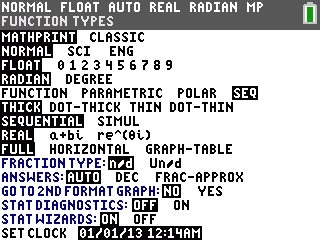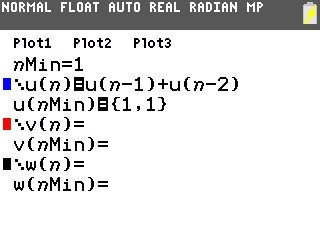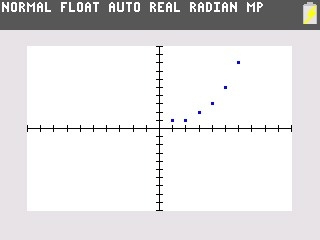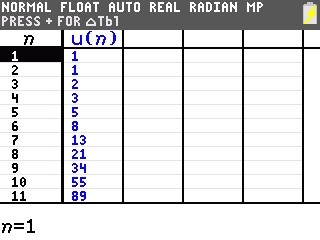# Knowledge Base

## Solution 34672: Graphing the Fibonacci Sequence on the TI-83 Plus and TI-84 Plus Family of Graphing Calculators.

### How can I graph the Fibonacci sequence on the TI-83 Plus and TI-84 Plus family of graphing calculators?

In a Fibonacci sequence the first two terms are 1 and 1. Each succeeding term is the sum of the two immediately preceding terms. Follow the procedure below to graph the Fibonacci sequence:

1) Press [mode]
2) Press [↓] [↓] [↓] [→] [→] [→] to select SEQ and press [enter]3) Press [Y=].
4) For nMin= press [↑]  [enter]
5) For u(n)= press [2nd]  [ ( ] [X,T,θ,n] [-]  [ ) ] [+] [2ND]  [ ( ] [X,T,θ,n] [-]  [ ) ] [enter]
6) For u(nMin)= press [2nd] [ ( ]  [,]  [2nd] [ ) ]7) Press [zoom] then  to select 6:ZStandard.8) To view the table press [2nd] [window] to setup the table options.
9) Press  [enter] to set the table to start at 1.
10) Press [2nd] [graph] to see the table of n and u(n).Please see the TI-83 Plus and TI-84 Plus Family guidebooks for additional information.

Last updated: 7/10/2023